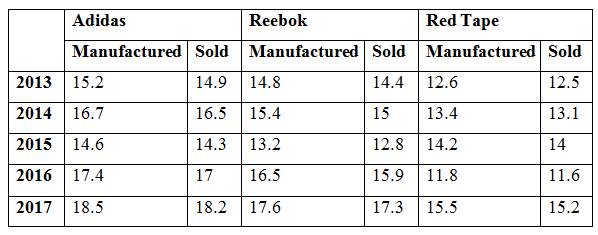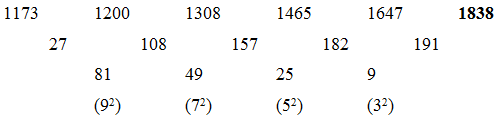# CWC/FCI Prelims 2019 – Quantitative Aptitude Questions (Day-19)

Dear Readers, Exam Race for the Year 2019 has already started, To enrich your preparation here we are providing new series of Practice Questions on Quantitative Aptitude – Section for CWC/FCI Exam. Aspirants, practice these questions on a regular basis to improve your score in aptitude section. Start your effective preparation from the right beginning to get success in upcoming CWC/FCI Exam.

[WpProQuiz 5607]

Directions (Q. 1 – 5) What value should come in place of (?) in the following number series?

1) 456, 228, 228, 456, 1824, ?

a) 14592

b) 9256

c) 12278

d) 13714

e) 10422

2) 1173, 1200, 1308, 1465, 1647, ?

a) 1916

b) 1792

c) 1945

d) 1873

e) 1838

3) 17, 20, 46, 147, 600, ?

a) 2740

b) 2575

c) 3015

d) 3260

e) 3420

4) 675, 674, 336, 111, 26.75, ?

a) 12.55

b) 4.35

c) 7.45

d) 5.25

e) 10.65

5) 124, 63, 71, 169, 740, ?

a) 5284

b) 4872

c) 3653

d) 6045

e) 4257

Directions (Q. 6 – 10) Study the following information carefully and answer the given questions:

The following table shows the total pair of shoes manufactured (In lakhs) and sold by different companies over the years.6) Find the difference between the total pair of shoes manufactured in the year 2013 to that of 2016 of all the given companies together?

a) 3.1 lakhs

b) 4.3 lakhs

c) 2.7 lakhs

d) 3.8 lakhs

e) None of these

7) Find the average pair of shoes manufactured by Adidas in all the given years together?

a) 15.62 lakhs

b) 14.26 lakhs

c) 17.54 lakhs

d) 16.48 lakhs

e) None of these

8) Find the ratio between the total pair of shoes unsold by Reebok in the year 2013, 2014 and 2015 together to that of total pair of shoes unsold by Red Tape in the year 2015, 2016 and 2017 together?

a) 18 : 5

b) 12 : 7

c) 23 : 11

d) 38 : 15

e) None of these

9) Total pair of Reebok shoes manufactured in all the given years together is approximately what percentage of total pair of Adidas shoes sold in all the given years together?

a) 84 %

b) 72 %

c) 96 %

d) 68 %

e) 112 %

10) Find the difference between the average pair of Reebok shoes sold in all the given years together to that of the average pair of Red Tape shoes sold in all the given years together?

a) 1.8 lakhs

b) 2.2 lakhs

c) 2.6 lakhs

d) 1.4 lakhs

e) None of these

Direction (1-5) :

The pattern is, *1/2, *1, *2, *4, *8,….The difference of difference is, 92, 72, 52, 32,….

The pattern is, *1 + 3, *2 + 6, *3 + 9, *4 + 12, *5 + 15,…

The pattern is,

(675 – 1)/1 = 674, (674 – 2)/2 = 336, (336 – 3)/3 = 111, (111 – 4)/4 = 26.75, (26.75 – 5)/5 = 4.35

The pattern is, *0.5 + 13, *1 + 23, *2 + 33, *4 +43, *8 + 53,….

Direction (6-10) :

The total pair of shoes manufactured in the year 2013 of all the given companies together

= > 15.2 + 14.8 + 12.6 = 42.6 lakhs

The total pair of shoes manufactured in the year 2016 of all the given companies together

= > 17.4 + 16.5 + 11.8 = 45.7 lakhs

Required difference = 45.7 – 42.6 = 3.1 lakhs

The average pair of shoes manufactured by Adidas in all the given years together

= > (15.2 + 16.7 + 14.6 + 17.4 + 18.5)/5

= > 82.4/5 = 16.48 lakhs

The total pair of shoes unsold by Reebok in the year 2013, 2014 and 2015 together

= > (14.8 – 14.4) + (15.4 – 15) + (13.2 – 12.8)

= > 0.4 + 0.4 + 0.4 = 1.2 lakhs = 120000

The total pair of shoes unsold by Red Tape in the year 2015, 2016 and 2017 together

= > (14.2 – 14) + (11.8 – 11.6) + (15.5 – 15.2)

= > 0.2 + 0.2 + 0.3 = 0.7 lakhs = 70000

Required ratio = 120000 : 70000 = 12 : 7

Total pair of Reebok shoes manufactured in all the given years together

= > 14.8 + 15.4 + 13.2 + 16.5 + 17.6 = 77.5 lakhs

Total pair of Adidas shoes sold in all the given years together

= > 14.9 + 16.5 + 14.3 + 17 + 18.2 = 80.9 lakhs

Required % = (77.5/80.9)*100 = 95.797 % = 96 %

The average pair of Reebok shoes sold in all the given years together

= > (14.4 + 15 + 12.8 + 15.9 + 17.3)/5

= > 75.4/5

The average pair of Red Tape shoes sold in all the given years together

= > (12.5 + 13.1 + 14 + 11.6 + 15.2)/5

= > 66.4/5

Required difference = (75.4/5) – (66.4/5) = 9/5 = 1.8 lakhs